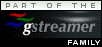# Introduction

The parser of Avisynth 3 will be able to parse an Avisynth script, similar to the 2.5 version. It will also add conditional (tests) and iterative (loops) structures.

A first version of the parser has already been implemented. But some problems raised, especially about the labels, used for loops. (detail a bit more).

Hence, the previous design has been abandoned and a second version is being written. It is composed of two levels:

• A low level parser, which mainly will allow to do computations on numbers and concatenate strings, call functions or manipulate the stack.
• A high level one, which will allow to write Avisynth scripts.

The parser has been written using the Spirit parser framework. It uses template meta-programming techniques. The expressions allow to approximate the syntax of Extended Backus Normal Form (EBNF) in C++.

That document is organized in three sections. First, the features of the parser. Then a description of each level of the parser is given, with a grammar overview, providing a formal definition of the grammar, a description of the virtual machine which evaluates the parsed data, and a description of the parser itself and on how the input data is used to generate the computational tree.

Table of content

# Low level parser

## Grammar

Here is the grammar of the low level parser in the EBNF syntax:

```top           ::= ( '!' >> lit | '@' functionTable | operators | stack )*
lit           ::= strict_real_p | int_p | stringLiteral | str_p("true") | str_p("false")
functionTable ::= ???
stringLiteral ::= lexeme_d [ '"' >> ( str_p("\\n") | ( anychar_p - '"' ) )* >> '"' ]
stack         ::= '[' >> int_p >> ']' >> ( ch_p('=') | eps_p )
```

'operators' are the following strings, with their meanings:

```"op+[ii]" : addition of two integers
"op+[dd]" : addition of two real numbers
"op+[ss]" : concatenation of two strings
"op-[ii]" : substraction of two integers
"op-[dd]" : substraction of two real numbers
"op*[ii]" : multiplication of two integers
"op*[dd]" : multiplication of two real numbers
"op/[ii]" : division of two integers
"op/[dd]" : division of two real numbers
```

We use the following Spirit primitives:

```strict_real_p : matches a real number (double) not being an integer.
int_p         : matches a signed integer (int) in base 10.
str_p         : matches a string literal.
ch_p          : matches a single character literal.
eps_p         : matches the NULL string.
lexeme_d      : turns off white space skipping.
anychar_p     : matches any single haracter, including '\0'.
```

With this grammar, you can do every mathematical operations involving numbers, like (3 + 2) * 5, or you can concatenate strings. You can manipulate the stack (saving and retrieving an element of the stack).

## Virtual machine

The virtual machine is basically a stack and a program counter. More precisely:

```std::deque<AVSValue> stack;
std::vector<Operation>::const_iterator pc;
```

The stack can manipulate booleans, integers numbers (int), real numbers (double), strings and clips.

The vector of Operation is built by the parser. An Operation is the following type:

```typedef boost::function< void (VirtualMachine&) > Operation;
```

The boost function is a function object wrapper. An Operation is then a function that returns nothing and that takes a reference of a VirtualMachine as argument.

The parser fills a vector of Operation objects. When the virtual machine runs, we have basically 4 possibilities:

1. If the parsed object is a "!lit" (see above), then its value is stored at the beginning of the stack of the virtual machine.
2. If it's an operation (+ ("op+[**]"), - ("op-[**]"), * ("op*[**]"), / ("op/[**]")), then the second element of the stack is changed in the operation of itself and the first element of the stack, and the first element of the stack is removed.
3. If it's a stack operation, you can save the element #n of the stack at the beginning of the stack ("[n]"), or retrivieng the first element of the stack, stores it in the element #n and remove it ("[n]=").
4. Todo: describe the "function" part

Let's take an example. We want to compute 7 * (2 + 3). The script is the following:

```!7 !2 !3 op+[ii] op*[ii]
```

The parser fill the vector of operations with basically:

```7, 2, 3, operator+, operator*
```

Then, the virtual machine runs, that is, we iterate over the vector of operations. Here are the steps:

1. It stores 7 at the first element of the stack (first column is the index of the stack element and second column is its value):
```0 7
```
2. It inserts 2 at the beginning of the stack.
```1 7
0 2
```
It inserts 3 at the beginning of the stack.
```2 7
1 2
0 3
```
3. It adds 3 to 2 (hence 5) by executing operator+ and stores the value in the second element.
```2 7
1 5
0 3
```
4. It suppresses the first element. The stack has then two elements:
```1 7
0 5
```
5. It multiplies 5 to 7 (hence 35) by executing operator* and stores the value in the second element:
```1 35
0 5
```
6. It suppresses the first element. The stack has finally one element:
```0 35
```
7. The iteration of the virtual machine is finished as the last element of the operations has been executed.

Note: The low level parser can do a segmentation fault (for example the string "!1 op+[ii]"). It is normal. It will be up to the high level parser to provide correct low level parser code.

# High level parser

## Parser